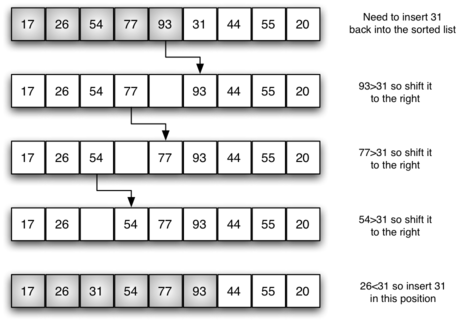## Data Structure : Binary Search Tree

Binary search trees (BSTs) are very easy to understand. Here we start with a root node n with value x, where the left subtree to n contains nodes with values < x and the right subtree contains nodes whose value are >=x. Here we are learn about Insertion into BST, Searching, Deletion from the tree and more about Binary Search Tree.
07, Aug, 2019 18:35 Created At 07, Aug, 2019
Created By Vijay Pal## Insertion Sort Algorithm | Complexity of Insertion Sort Algorithm

Insertion sort is a simple sorting algorithm that works the way we sort playing cards in our hands. This is an in-place comparison-based sorting algorithm. In this article we are going to learn algorithm of Insertion Sort, What is the time complexity of Insertion Sort, and How we can calculate it?
26, Jul, 2019 14:50 Created At 26, Jul, 2019
Created By Vijay Pal## Bubble Sort Algorithm | Complexity of Bubble Sort Algorithm

Bubble Sort is a simplest sorting algorithm, that works on repeatedly swapping adjacent elements if they in wrong order. In this article we are going to learn algorithm of bubble sort, example of bubble sort, and how to calculate time complexity of Bubble Sort.
26, Jul, 2019 14:50 Created At 26, Jul, 2019
Created By Vijay Pal## Merge Sort Algorithm | Complexity of Merge Sort Algorithm

In this article we are going to learn Merge Sort algorithm and its implementation. And also we are describe how to calculate complexity of Merge Sort in form T(n) and Big Oh O(n log n).
26, Jul, 2019 14:50 Created At 26, Jul, 2019
Created By Vijay Pal

## Find All Pair of Elements whose Sum is Equal to K

Write a program to find all pair element of given array whose sum is equal to given value K.
26, Jul, 2019 14:49 Created At 26, Jul, 2019
Created By Vijay Pal